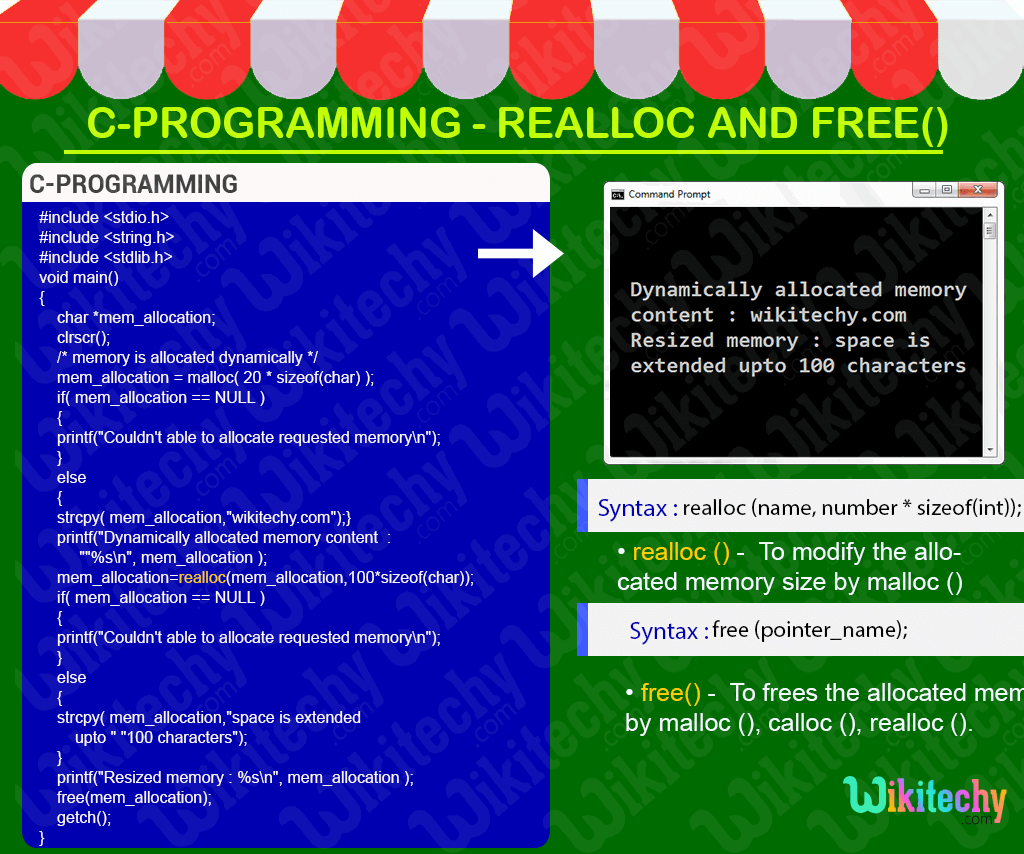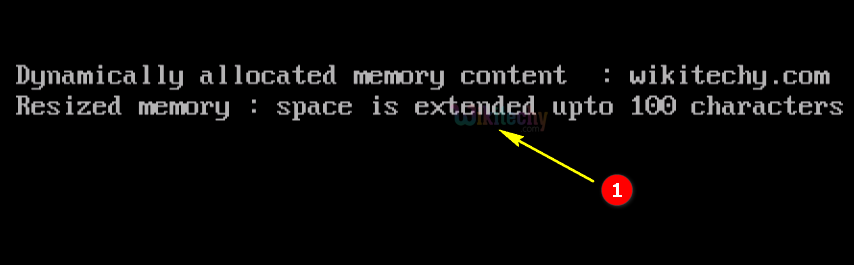# realloc & free in C

## Structure and Linked List with C Programming in TamilLearn C - C tutorial - realloc - C examples - C programs

## C - realloc() & free() Function - Definition and Usage

• In C- Programming the realloc () function is used for modifying the allocated memory size by malloc () and calloc () functions to new size.
• In C-Programming the realloc function is used for extending the allocate memory space.## C Syntax## Free() Function :

• In C-Programming the free function is used to frees the allocated memory by malloc (), calloc (), realloc () functions and returns the memory to the system.## C Code - Explanation1. In this statement we assign the address of the “q” variable to “ptr” .
2. In this printf statement we print the address of the variable “q” .
3. In this printf statement we print the value of the variable “q” .
4. In this statement the memory space will be allocated where the content will be assigned for the certain variable.
5. In this statement we reallocate the memory space where we extend the memory space using realloc function.
6. In this statement we check the memory space is empty are not.
7. In this printf statement we print the extended memory space.

## Sample Output - Programming Examples1. In this statement we declare the pointer variable “mem_allocation” as character datatype.
2. In this statement we allocate the memory space dynamically using calloc function.
3. In this condition we check the memory space is empty or not..

## Sample Code